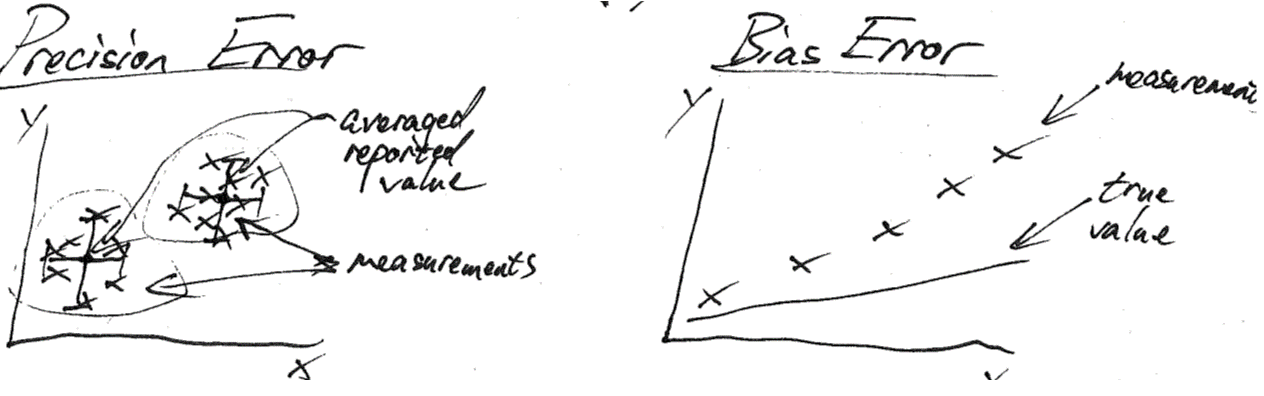Now that we’ve covered the design/layout, procedure, and instrument calibration and traceability for our experiment, it’s time to start analyzing our data.

Section 4.3: Data Analysis and Uncertainties

The goals for this section of your report are

1. Show us what happens to a raw data point prior to being reported, such that the raw data can be analyzed by someone, somewhere else.
2. Show us where uncertainties of the reported values come from (i.e. bias error, precision error, etc.)
3. Quantify how confident you are in the reported measurements.
4. Conform to ASTM Standard E2586 – Standard Practice for Calculating and Using Basic Statistics. You can download the pdf for free while on campus.

How do we quantify confidence? This is where we realize the value of our engineering education. We’ve all calculated a standard deviation by now and know that 68% of the measurements fall within ±σ of the mean (a.k.a. a coverage factor of 1), 95% lie within ±2σ (a.k.a. a coverage factor of 2), and 99.7% lie within ±3σ (a.k.a. a coverage factor of 3). While it’s straight forward to calculate the coverage factor for every steady state measurement you take. You should also do a repeatability test at several points to double check. A classic example is that someone states that there uncertainty has a coverage factor of 2 (99.7% of all measurements fall with y of mean), however this is only a repeatability test of the precision error. You still need to propogate instrument uncertainties to determine the bias error.This portion of the lesson contains a considerable amount of math as we go through the Root-Sum-Square (RSS) method and what it means for uncertainty propogation. Remember that we can do this math by hand or with EES, which will estimate the uncertainties numerically. Here’s a link to the hand-written notes: lesson-6-lecture-notes.